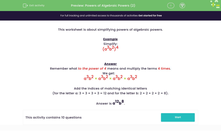# Understand How to Multiply Algebraic Powers

In this worksheet, students will simplify powers of algebraic powers by multiplicationKey stage:  KS 3

Curriculum topic:   Algebra

Curriculum subtopic:   Simplify Algebraic Expressions to Maintain Equivalence

Popular topics:   Algebra worksheets

Difficulty level:#### Worksheet Overview

This activity is about simplifying algebraic powers by multiplication.

Example

Simplify:

(a3b2)4

Remember what to the power of 4 means and multiply the terms 4 times.

We get:

a3b2 × a3b2 × a3b2 × a3b2

Add the indices of matching identical letters

(for the letter a: 3 + 3 + 3 + 3 = 12 and for the letter b: 2 + 2 + 2 + 2 = 8).

Now that you can see what is actually happening, we can use a quicker method to work these out.

All we do is to multiply the powers inside the bracket with the power outside the bracket.

a has the power of 3, so we multiply 3 by 4: 3 x 4 = 12

b has the power of 2, so we multiply 2 by 4: 2 x 4 = 8

a12b8.

Let's have a go at some questions.

### What is EdPlace?

We're your National Curriculum aligned online education content provider helping each child succeed in English, maths and science from year 1 to GCSE. With an EdPlace account you’ll be able to track and measure progress, helping each child achieve their best. We build confidence and attainment by personalising each child’s learning at a level that suits them.

Get started